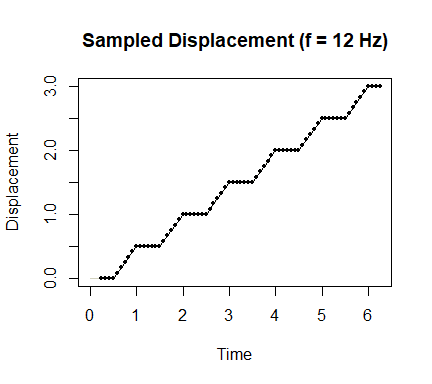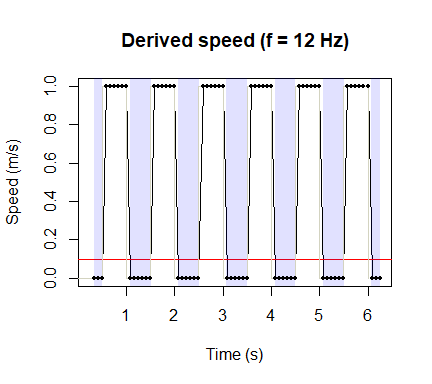# Detecting stop-start motion in trajectories

#### 2023-06-24

If a trajectory contains periodic stop-start motion, what sampling frequency is required to detect it? A first assumption might be that the Nyquist rate is adequate. However, that is not the case, since when we work with trajectories, we are not measuring speed directly, but instead approximating it by measuring the mean speed between pairs of points.

To see why, let’s use an example. We will simulate a trajectory of an animal that repatedly stops for 0.5 secs then walks at 1 m/s for 0.5 seconds, so speed forms a square wave with frequency 1 Hz. We will will consider any speed slower than 0.1 to be stopped.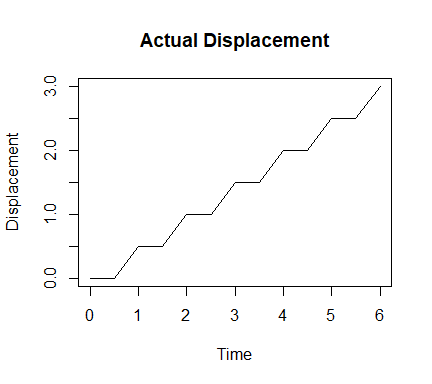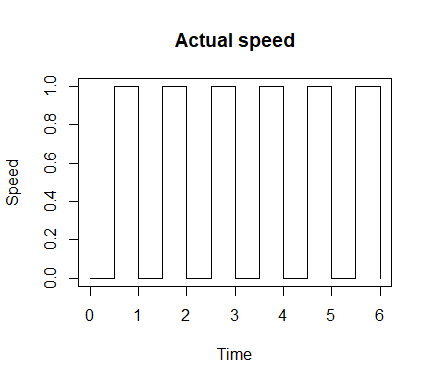If we create a trajectory by sampling at the Nyquist rate of signal frequency * 2 = 2 Hz, this is the result, which looks good: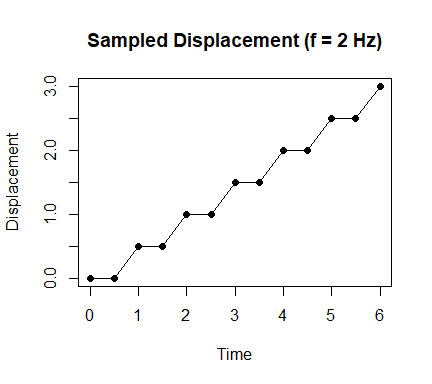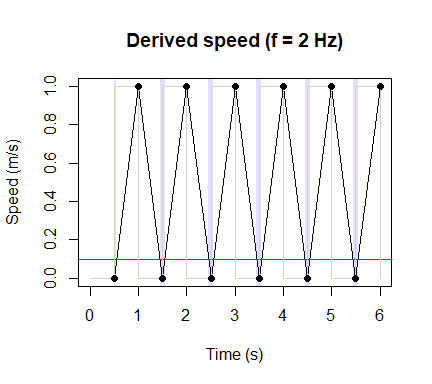But it turns out we were “lucky” here, and this frequency is only adequate if the sampling is in phase with the trajectory. If we phase shift the sampling times by 1/4 of the signal wavelength without altering the sampling rate, we suffer from signal aliasing and no longer detect any variation in speed! Remember that we are actually sampling displacement, and then deriving speed from the sampled displacement.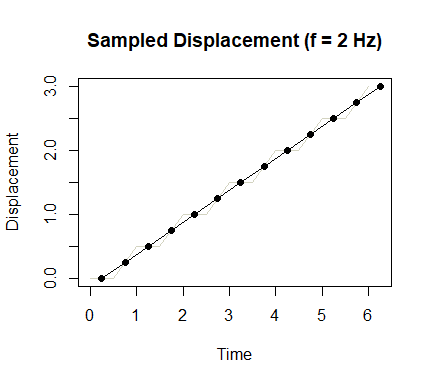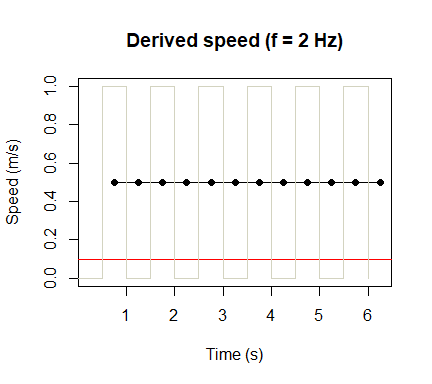To reliably detect stopping, we need to sample (at least) twice within a stopped (or moving) period. In our example, the animal stops for 0.5 seconds at a time. To ensure we sample stopped periods twice regardless of phase shifts, we need to sample at a minimum frequency of 3 / stopping duration = 6 Hz. Now we are certain to obtain the correct frequency, regardless of phase shifts in sampling, although stopping and moving durations and acceleration are still very inaccurate.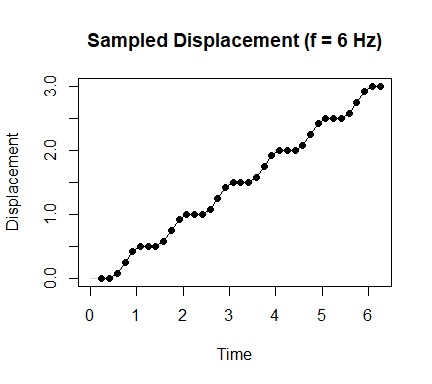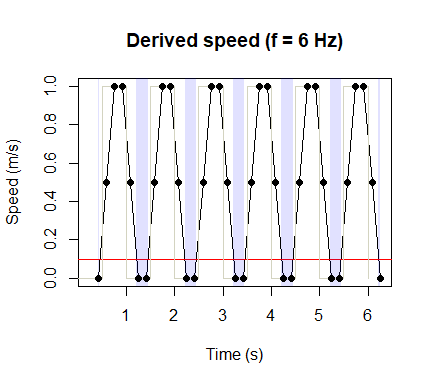Furthermore, if the speed is actually closer to a sine wave than a square wave, detecting stop-start motion is harder still.

## Conclusion

If you are trying to detect the frequency of stop-start motion in a trajectory, the highest frequency that it is theoretically possible to detect reliably is 1/4 the sampling frequency. However, what actually matters is the number of samples within a stopped or moving period, rather than the frequency of stopping and starting. So let’s just sample at a very high frequency!